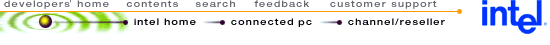## Using MMX™ Instructions for 3D Bilinear Texture Mapping

 Disclaimer Information in this document is provided in connection with Intel products. No license, express or implied, by estoppel or otherwise, to any intellectual property rights is granted by this document. Except as provided in Intel's Terms and Conditions of Sale for such products, Intel assumes no liability whatsoever, and Intel disclaims any express or implied warranty, relating to sale and/or use of Intel products including liability or warranties relating to fitness for a particular purpose, merchantability, or infringement of any patent, copyright or other intellectual property right. Intel products are not intended for use in medical, life saving, or life sustaining applications. Intel may make changes to specifications and product descriptions at any time, without notice. Copyright © Intel Corporation (1996). Third-party brands and names are the property of their respective owners.

Summary

This application note presents an optimized MMX(TM) technology texture mapping algorithm, using bilinear interpolation as a filter and quadratic approximation for perspective correction. Background and a brief description appear in sections 1 and 2. Sections 2-4 describes the flow of the code. Peformance analysis is given in section 6, proving the MMX code about 5x faster than optimized C code. Complete inner-loop source code is given in the Appendices, but the user will need to create an application around this inner loop. Note that bilinear interpolation is a high-quality data-intensive algorithm; simpler methods like linear interpolation or point-sampling will yield higher pixel rates but lower quality.

1.0 Introduction

The Intel Architecture MMX(TM) technology extensions use a Single Instruction Multiple Data (SIMD) technique to speed up software, by processing multiple data elements in parallel. This application note illustrates how to use the SIMD techniques to achieve better performance in filtered 3D graphics texture mapping. Texture mapping is a process of assigning a texture bitmap coordinate to a screen coordinate as shown in Figure 1.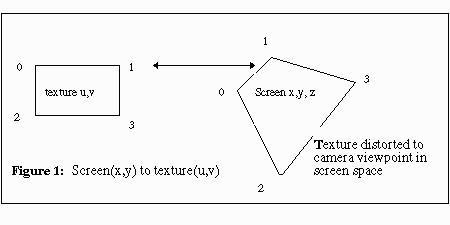This code does perspective texture mapping with Bilinear Interpolation for filtering and Quadratic Approximation for perspective correction. Figure 2 shows where the algorithm fits in a typical 3D rendering engine.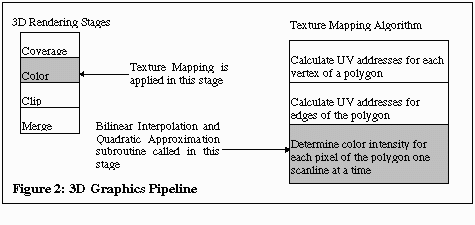2.0 The Bilinear Interpolation Algorithm

Bilinear interpolation is a filter for texture mapping. Texture mapping based on a simple “point sampling” algorithm samples one texture coordinate for each pixel coordinate, as shown in Figure 3. But that can yield very “blocky” or “pixellated” results, especially when the texture is seen close up (magnified) on the screen. For smoother appearance, bilinear interpolation samples 4 texture pixels, taking a weighted average to produce each pixel on the screen.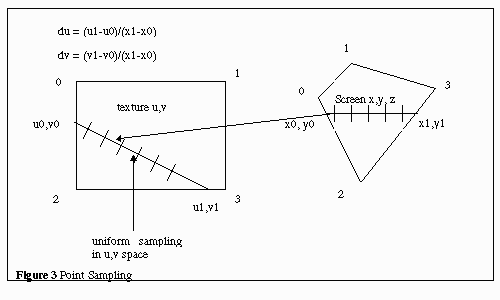Given a screen pixel point and a texel coordinate (U.u,V.v), the algorithm finds 4 adjacent points on the texture map and interpolates the RGB color intensity for the pixel based on the colors of the 4 points. The RGB color intensity is calculated by multiplying the four points by a weighting factor(equations 1-4) and adding the results, as shown in Figure 4.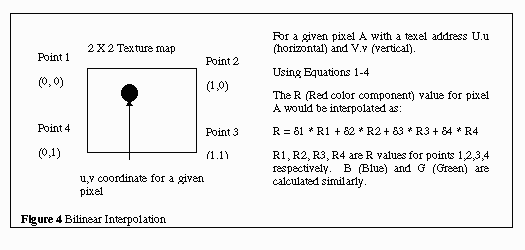The algorithm is given a U.u,V.v coordinate, which contains a integer part and a fractional part. The integer part is extracted to obtain the first point in the texture, (U, V). The 3 adjacent points are (U+1, V), (U,V+1), (U+1, V+1). The actual (U.u,V.v) point is located somewhere within these 4 points. The RGB values of each of these four points are read, and the RGB value for the corresponding pixel is interpolated from these four points and the fractional parts.

The fractional part of the U.u,V.v coordinate is used to calculate the weight factor for each of the 4 points. Let the fractional part of U.u be .u and the fractional part of V.v be .v. Let (U, V), (U+1), (U+1, V+1) and (U, V+1) be point1, point2, point3, and point4 respectively, and let ð1, ð2, ð3 and ð4 the weight factor for points 1 through 4 respectively. Then the equations for the four factors are given below:

 ð1 = (1- .u) · (1 - .v) (1) ð2 = .u · (1 - .v) (2) ð3 = .u · .v (3) ð4 = (1 - .u) · .v (4)

The new RGB value for the pixel being mapped is given by the equations below:

 R = R1 · ð1 + R2 · ð2 + R3 · ð3 + R4 · ð4 (5) G = G1 · ð1 + G2 · ð2 + G3 · ð3 + G4 · ð4 (6) B = B1 · ð1 + B2 · ð2 + B3 · ð3 + B4 · ð4 (7)

where Rx, Gx and Bx are RGB values for point x, where x can be 1, 2, 3, and 4.

Quadratic Approximation is used in perspective texture mapping to eliminate distortions in mapped images. For a scanline, the first u,v point is given and the du, dv, ddu, ddv values are given to calculate the next u,v point. The du and dv parameters are texture slopes, and they determine how UV addresses change from one pixel to the next. ddu and ddv are the slopes of du and dv. The height and length of a 3D object become compressed(appear shorter) as it goes away from the viewer. The UV address will be changed in larger steps as the polygons move away from the viewer. For true perspective correction of the texture, two divisions by W are needed for each pixel as shown in Figure 5. (W is the world space coefficient, derived from the 4x4 matrices used to transform each vertex from its location in its local “model” space to the camera viewpoint in screen space). Quadratic approximation is used to approximate the “1/W” effect, avoiding the division operations. By quadratic approximation, we mean addition of a delta to U & V at each screen horizontal scanline step, and addition of a second-order differential to the delta factors.

The second-order differentials, ddu and ddv, approximate the 1/W division. This algorithm is given by the equations below:

 ui+1 = ui + dui (8) vi+1 = vi + dvi (9) dui+1 = dui + ddu (10) dvi+1 = dvi + ddv (11)

For background information on calculating the quadratic values ddu and ddv, and their derivation from 1/W, refer to "Digital Image Warping" by George Wolberg, pg. 297 Appendix 3.[ IEEE Computer Society Press, July 1990. ISBN 0-8186-8944-7]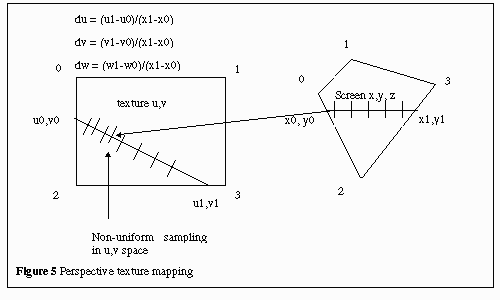3.0 Input and Output Data Representation

The intensity of a pixel is represented by a 16-bit WORD in which the RGB is stored in a 565 data format: 5 bits for R, 6 bits for G and 5 bits for B. However, the algorithm can be easily modified for 555 color format. The color of a texel is represented by a byte unsigned number. The byte is an index to the color palette entry, where the actual color intensity is stored. Each color palette is represented by a 32-bit DWORD, in which the RGB values, are stored in the lowest 24 bits. A 24-bit color palette is used instead of 565 RGB format because color calculation is done in bytes or words. The (U.u,V.v) coordinates of a texture map are represented by 32-bit DWORD, in which the value is a 16.16 number(16 bit integer part and 16 bit fractional part). The du, dv, ddu, and ddv values also share the same data format. Refer to Figure 6 and 7 for further clarification.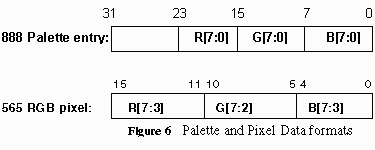The ranges of R,G and B values and the color palette index are from 0 to 255. The 16 bit fractional part of the u,v values will be truncated to 15 bit for signed multiplication. The texture map must be square, with each side 0 <= u, v < 2N, where 2N is the size of the texture. U and V must be values between 0 and 255.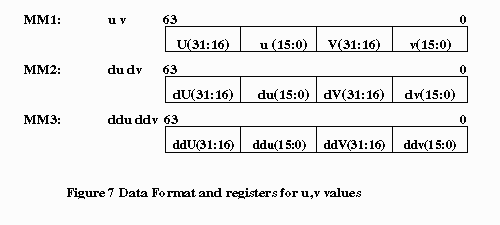Each value is a 16.16 DWORD, with U & V unsigned, and du, ddu, dv, and ddv signed.

4.0 Code Partitioning

The procedure is composed of two sections: data setup, and inner loop. The data setup section copies the data pointers to the general purpose registers and copies the u,v, du,dv,ddu and ddv values to the MMX registers. The inner loop section consists of setting up data for parallel multiplication and addition, manipulating the integer and fractional part of the (u,v) coordinate, calculating the corresponding pixel intensity, and writing the result to memory.

4.1 Data Setup

There are three tasks in data setup: move texel map pointer and palette pointer to EAX and ECX respectively, and unpack u, v, du, dv, ddu and ddv values to MM1, MM2 and MM3 respectively. Five unpack instructions are used to arrange the data.

4.2 Inner Loop Section

The inner loop performs the bulk of the bilinear interpolation and quadratic approximation. There are 6 major tasks: Calculating the 4 ð multipliers, calculating the integer (U.u,V.v) which references the linear memory array of the texture map, reading the 4 palette indices (texels) and corresponding RGB pixels from the palette, arranging them in the proper format, calculating the new RGB intensity for the screen pixel, and finally calculating the next (U.u,V.v) point.

4.2.1 Calculating the 4 ð multipliers

This is the most challenging part of the code in terms of the number of instructions required. First there are accuracy versus instruction count issues. The algorithm requires calculating (1 - .u) and (1 - .v), which is the same as taking the 2’s complement of both values. However, if .u or .v were 0, the code needs to produce a result of 1. The multipliers, however, are fractional fixed point values. In order to handle this special case, there would be added instructions and conditional statements. Taking the 1’s complement of .u and .v to approximate (1 - .u) and (1 - .v) is the better solution; although it will lose one bit of accuracy. In cases where .u and .v equals 0 or 0.5, the final result is accurate to within one lsbit. This is tolerable since the fractional part is truncated to 16 bits anyway, and the algorithm only uses the integer part of the result.

Due to the way the pixels are read into the registers, the ð multipliers are arranged in the format shown below:

MM1: ð4 ð1 ð4 ð1

MM2: ð2 ð3 ð2 ð3

MM3: ð4 ð1 ð2 ð3

This arrangement is used to speed up the RGB color calculation. pmulhw is used to obtain the high word as the final result. Figure 8 below illustrates the code. NOTE: the example code below is not optimized for pairing, but for ease of reading; however, the full code listings in the appendices are optimized.

 .DATA reg_clr5     DWORD      0000ffffh,      0000ffffh reg_xoru1    DWORD      7fff7fffh,      7fff7fffh reg_xorv1    DWORD      00007fffh,      00007fffh reg_xorv2    DWORD      7fff0000h,      7fff0000h .code pand         mm0,  reg_clr5 ;  clear out integer part psrld        mm0, 1 ;  shift u,v 1 bit for sign multiply movq         mm4, mm0 ;  make a copy for the other operand punpcklwd    mm0, mm0 ;  mm0 = - - u u punpckhwd    mm4, mm4 ;  mm4 = - - v v punpckldq    mm0, mm0 ;  mm0 = u u u u punpckldq    mm4, mm4 ;  mm4 = v v v v movq         mm3, mm4 ;  mm3 = v v v v pxor         mm4, reg_xorv2 ;  1's complement mm4 = ~v v ~v v pmulhw       mm4, mm0 ;  mm4 = ð2 ð3 ð2 ð3 movq         mm7, mm4 ;  mm7 = ð2 ð3 ð2 ð3 pxor         mm3, reg_xorv1 ;  1's complement mm3 = v ~v v ~v pxor         mm0, reg_xoru1 ;  mm0 = ~u ~u ~u ~u pmulhw       mm3, mm0 ;  mm3 = ð4 ð1 ð4 ð1 punpckldq    mm4, mm3 ;  mm4 = ð4 ð1 ð2 ð3 Figure 8: Implementation of ð multiplier

4.2.2 Calculating the Integer Part of U,V

This section explains the steps in calculating the integer part of the (U.u,V.v) coordinate. (U, V) is used to reference the palettized 8-bit texel stored in the texture map. The texture map is a 256X256 array (or 64K linear memory segment), where each entry is 8 bits wide. Hence, the displacement in the texture map for a given (U, V) coordinate is U+V*256. This references point 1 in the texture map. Let’s call this point pt1. (If the texture map were instead 16 or 24 bits/pixel, then the V-multiplier would need to be scaled accordingly by another factor of 2 or 3.)

Point 2 in the texture map is then pt1 + 1, point 3 is pt1 + 256 and point 4 is pt1 + 257. There is also one special case to consider when the 3 adjacent points go out of the 256 X 256 frame. This implementation uses tiling to solve this problem. Tiling simply wraps the number around when any of the points go out of the 256 X 256 frame. For example, a point with u= 256 and v=256 is the same as a point with u=0 and v=0. This code is given in Figure 9.

An alternative to tiling is to wrap-around to the next texture scanline when at the right edge of the texture. However, it reduces quality and it only saves 4 instructions, or 2 cycles when paired, for the 256x256 texture-size hardwired case.

The texels are read in the following order: pt1, pt4, pt3, pt2. This particular order is chosen to minimize the number of instructions required to calculate the pointers to the four texel points.

 .DATA reg_clr6        DWORD 00ffh,           00ffh ; calculate index in linear texel map array based on U & V psrld mm7, 16 ; get integer part of U.u and V.v pand  mm7, reg_clr6 ; allow only 256x256 texture size movq  mm6, mm7 ; mm6 = mm7 = 0 V 0 U (integer part) psrlq mm6, 24 ; mm6 = 0 0 0 [V*256](shifted     24=32-8places) paddd mm6, mm7 ; mm6 = 0 V 0 [U+V*256] movd  eax, mm6 ; move index to eax to get point 1 mov   ebx, eax ; copy index value ; read in texel and color one texel at a time xor   edx, edx ; used to prevent Pentium Pro         processor stalls mov   dl, [esi][eax] ; get color pallette pointer of          point 1 movq  mm5, [edi][edx*4] ; get RGB of point 1 from color     pallete ; mm5 = - - - - 0 R1 G1 B1 add   eax, 256 ; calculate point 4 index and   eax, 0ffffh ; take modulo 256x256 xor   edx, edx ; mov   dl, [esi][eax] ; get color pallette index of     point 4 punpcklbw mm5, [edi][edx*4] ; get RGB of point 4 from color       pallete ; mm5 = 0 0 R4 R1 G4 G1 B4 B1 add   ebx, 1 ; calculate point 3 index and   ebx, 0ffh ; take modulo 256 and   eax, 0ff00h ; retain only y component or    eax, ebx ; linear address calculation xor   edx, edx ; mov   dl, [esi][eax] ; get color pallete index of point 3 movq  mm6, [edi][edx*4] ; get RGB of point 3 from color     pallete ; mm6 = - - - - 0 R3 G3 B3 add   eax, 0ff00h ; calculate point 2 index and   eax, 0ffffh ; take modulo 256x256 xor   edx, edx ; mov   dl, [esi][eax] ; get color pallete index of point 2 punpcklbw mm6, [edi][edx*4] ; get RGB of point 2 from color     pallete ; mm6 = 0 0 R2 R3 G2 G3 B2 B3 Figure 9: Texture map displacement calculation

4.2.3 Reading Four RGB intensity values

Code for reading color values is intermixed with the (U,V) coordinate calculations (Figure 9). Each texel is read immediately after the texel address is known. Two 24-bit texels are packed into one MMX register as each is read from the palette (Figure 10). This arrangement facilitates parallel PMADD instructions, as explained in the next section, and reduces the number of registers to temporarily store these values.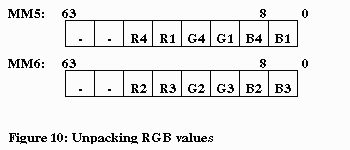4.2.4 Calculating the RGB intensity for the pixel

This part calculates the final texel result to write to the output buffer. The ð multipliers are stored in available MMX registers in the format as discussed in section 4.2.1. Figure 11 illustrates the flow of the data.

The code for the calculation is given in Figure 12. The MMX registers used here are different from the actual code for the sake of clarification. Because of the way the RGB values are arranged, we are able to perform multiply and add on two pixels in parallel.The “R” is calculated by further unpacking MM0 and using one more addition. The final “G” and “B” value are calculated with just one more addition by adding MM5 and MM6. The final RGB format can be obtained using “shift” and “or” instructions.

 ; arrange RGB values in proper format for parallel multiply xor          mm7, mm7 ; mm7 = 0 0 0 0 movq         mm3, mm5 ; mm3 = mm5 = 0 0 R4 R1 G4 G1 B4 B1 punpcklbw    mm5, mm7 ; mm5 = 0 G4 0 G1 0 B4 0 B1 movq         mm0,  mm6 ; mm0 = mm6 = 0 0 R2 R3 G2 G3 B2 B3 punpcklbw    mm6, mm7 ; mm6 = 0 G2 0 G3 0 B2 0 B3 punpckhwd    mm0, mm3 ; mm0 = 0 0 0 0 R4 R1 R2 R3 punpcklbw    mm0, mm7 ; mm0 = 0 R4 0 R1 0 R2 0 R3 pmaddwd      mm5, mm1 ; mm5 = G41 B41 -- mm1 = ð4 ð1 ð4 ð1 pmaddwd      mm6, mm2 ; mm6 = G23 B23 -- mm2 = ð2 ð3 ð2 ð3 pmaddwd      mm0, mm3 ; mm0 = R41 R23 -- mm3 = ð4 ð1 ð2 ð3 punpckldq    mm4, mm0 ; mm4 = R23 - paddd        mm5, mm6 ; mm5 = G B paddd        mm0, mm4 ; mm0 = R - Figure 12: RGB intensity calculation

4.2.5 Final RGB data format

The final required data format as required by different video cards or software can be easily obtained with a few more MMX instructions. This code implements the 565 format as shown in Figure 6 and Figure 13 below. But 555 or 24(888) bit color can also be easily obtained, with 24 bit color requiring the fewest instructions.

 ; converts 24 bit color to 16 bit color pand       mm5, reg_clr2 ;  clear out irrelevant bits(G and B) ;  2 relevant bits are in lower word ;  due to ð multipliers shifted by 2      bits pand       mm0, reg_clr3 ;  clear out irrelevant bits(Red) punpckhdq  mm7, mm5 ;  mm7 = G - psrld      mm5, 17 ;  B is correct position psrlq      mm7, 43 ;  G is correct position por        mm5, mm7 ;  B with G psrlq      mm0, 38 ;  R in correct position por        mm5, mm0 ;  final result in mm5: 565 RGB format Figure 13: 565 data format manipulation

This implementation requires only 2 lines of code in the inner loop.

 paddd      mm1, mm2 ; performs u = u + du, v = v + dv paddd      mm2, mm3 ; performs du1 = du0 + ddu and     dv1 = dv0 + ddv Figure 14: Quadratic Approximation Implementation

5.0 Three versions of code

There are three different versions of the texture mapping code:

1: Optimized with MMX technology. It supports only 256X256 texture size.
2: Scalar C version, for performance comparison and functional description. NOTE THAT this C code is NOT OPTIMIZED.
3: A more flexible implementation with MMX technology. Allows user-specified texture sizes: 16x16, 32x32, 64x64, 128x128, or 256x256. Two more input parameters are required for this version. Because of the added instructions, the third version requires 4 more cycles.

All three versions of the code are given in the appendices.

There were three major design issues made to optimize for performance. Additional instructions were added to eliminate partial stalls on the Pentium(R) Pro processor. Accuracy was compromised for speed. Loop unrolling was not used, thus avoiding added complexity and more instructions.

XOR instructions are added to eliminate “partial-stalls” on the Pentium Pro processor, as shown in Figure 9. On that processor, accesses to a 32-bit register(EAX, EBX, ECX, EDX, etc...) after some previous instruction wrote to a partial register(AL, AH, AX, etc...) causes a partial-stall penalty of more than 6 clocks (the execution stops until the 16-bit or 8-bit partial-register update completes). By adding an XOR instruction “XOR EAX, EAX”, as recommended by VTune 2.0, the partial-stall caused by a byte update of AL is avoided. This added a total of 4 instructions in the inner loop, but it saved 24 penalty cycles.

As described earlier, a 1’s complement was used to approximate two’s complement (1- .x) numbers. Although one digit of accuracy is lost, it saves 2-4 instructions per subtraction.

There is one 16-bit write per iteration, of the RGB value to the screen. It would be possible to unroll the loop 4 times to take advantage the movq instruction to store 8 bytes to memory each time. However, the overhead might actually slow down the code. To implement loop unrolling, we have to consider the variations in the number of pixels per scanline. This requires conditional statements, which can cause branch mispredictions due to the irregularity of the pixel counts in a scanline. Note that the Pentium(R) Pro processor stalls 12 cycles for a branch mispredict

7.0 Performance

The optimized code has 103 instructions. With pairing, it requires 73 cycles to execute assuming an infinite cache. The inner loop requires 47 cycles. With a 16K L1 data cache, the frequency of cache hit per scanline depends on how the du, dv, ddu, and ddv vary and on the size of texture map. For example, du=1, dv=0 will sequentially fetch texels from a single cache line, with very good performance; but du>31 and/or dv>0 will skip across multiple cache lines at each texel fetch. Large (such as 256x256 byte = 64kB total) textures will have poorer L1 cache hit ratios than smaller textures(such as 32x32 = 1kB).

The number of pixels per scanline determines how many times the inner loop executes. The inner loop has four byte-sized reads (8-bit texture map entries) and four DWORD reads (color palette). The regularity in locality of reference of the texture map depends on the geometry and orientation of the rendered object. The four references to the color palette do not have any locality. However, since the color palette has only 256 entries of four bytes each, the entire palette has a good chance of being in the cache most of the time.

The table below gives lists the number of clocks required to map a given a scanline based on the number of pixels per scanline. Note that clock counts increase linearly with the number of pixels, due to the small overhead required per scanline. A total of 16 clocks are required in the outer loop, independent of the pixel count per scanline.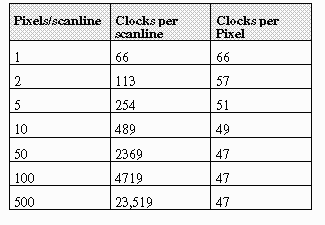Please reference the Table below for a comparison and performance analysis of the MMX technology and scalar implementation of texture mapping using bilinear interpolation and quadratic approximation. By commenting out the updates of ddu and ddv in the code, the reader can determine the (miniscule) performance impact and (significant) quality impact of non-perspective-correct mapping.

The executables for the two applications were compiled with Microsoft Visual C++ 4.1, with speed optimization turned on. Timings were measured with Intel’s VTune 2.0. [see http://www.intel.com/ial/vtune for information on ordering this product.]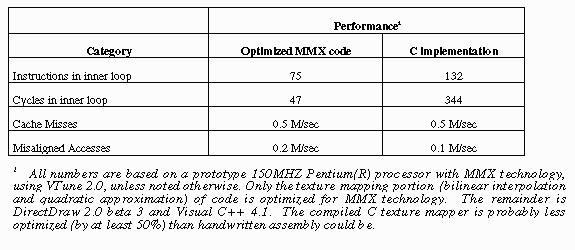8.0 Extrapolations

We leave as an excercise for the reader, the following worthwhile activities:

· Adaptation to RGB24 and/or RGB555 output pixel formats.

· Adaptation to RGB24 and/or RGB555 or RGB565 textures (no palette).

· Transparent, blended, or chromakeyed textures

· Fog

9.0 Tidbits

Below is a list of tips learned from writing this code:

· Reduce the number of pack and unpack instructions where possible. Shift and pack instructions share one shifter, so they    can’t be paired.

· Given two instructions that are pairable(one of the instruction can be issued to the V-pipe and the other can only be issued to    the U-pipe), schedule the one that requires the U-pipe first. Since the first instruction is always issued to the U-pipe first on    the Pentium processor, the second instruction can’t be paired if it requires the U-pipe.

· PMADD and PMUL each require 3 cycles. Schedule these instructions 3 cycles ahead before you reference the result.

· The data in an integer register must be ready two cycles before it is used by an MMX instruction as an address to reference    memory.

· Try to reuse MMX registers, since there are only eight.

APPENDIX A: Optimized MMX(TM) Code

The following is the optimized MMX code that implements Bilinear Interpolation with Quadratic Approximation for perspective correction. It assumes a fixed 256X256 texture. Note that “NOP” instructions are inserted to guranteed appropriate pairing in subseqent instructions. NOPs do not add more cycles, since they are paired with other instructions.

; prevent listing of iammx.inc file
.nolist
INCLUDE iammx.inc ; MMX technology assembly Macros

.486P
.model FLAT

;****************************************************************************
; Data Segment Declarations
;****************************************************************************
.DATA
reg_clr2     DWORD      003e0000h,    003f0000h
reg_clr3     DWORD      00000000h,    003e0000h
reg_clr4     DWORD      0000fc00h,    0f800f800h
reg_clr5     DWORD      0000ffffh,    0000ffffh
reg_clr6     DWORD      000000ffh,    000000ffh
reg_xoru1    DWORD      7fff7fffh,    7fff7fffh
reg_xorv1    DWORD      00007fffh,    00007fffh
reg_xorv2    DWORD      7fff0000h,    7fff0000h
reg_dduv     DWORD      00000000h,    00000000h
reg_blnk     DWORD      0h,   0h

;****************************************************************************
; Constant Segment Declarations
;****************************************************************************
.const

;****************************************************************************
; Code Segment Declarations
;****************************************************************************
.code
COMMENT ^
int32 *texel_ptr,
int32 *dest_ptr,
int32 *color_pal,
int32 num_of_pixels,
int32 u,v,du,dv,ddu,ddu);
^

bilinear_quadratic PROC NEAR C USES edi esi ebx,

texel_ptr:PTR SDWORD, dest_ptr:PTR SDWORD,
color_pal:PTR SDWORD,
num_of_pixels:DWORD, u:DWORD,
v:DWORD, du:DWORD, dv:DWORD,
ddu:DWORD, ddv:DWORD

 ; move commonly used pointers to registers/initialization movq       mm1, u ;  copy v coordinate to mmx register punpckldq  mm1, v ;  copy u/v in mm1 register (64 bits) movq       mm2, du ;  copy dv value to mmx register movq       mm7, mm1 ;  copy u/v for int. part conversion punpckldq  mm2, dv ;  copy du/dv into one mmx register movq       mm3, ddu ;  copy ddv value to mmx register psrld      mm7, 16 ;  get integer part of u and v punpckldq  mm3, ddv ;  copy ddu/ddv to one mmx register movq       mm6, mm7 ;  mm6 = mm7 = 0 v 0 u (int. part) mov        ecx, dest_ptr ;  move image pointer to ecx psrlq      mm6, 24 ;  multiply u and v by 256 movq       reg_dduv, mm3 ;  reg_dduv = ddv ddu paddd      mm6, mm7 ;  mm6 = 0 [u+v*256] mov        esi, texel_ptr ;  move texture image pointer to reg. movd       eax, mm6 ;  copy index to get point 1 Note mm6 is      free movq       mm0, mm1 ;  make another copy of u/v for mod. mov        edi, color_pal ;  move color palette base pointer to      register mov        ebx, eax ;  copy index value paddd      mm1, mm2 ;  performs u = u + du and v = v + dv add        eax, 256 ;  calculate point 4 index paddd      mm2, reg_dduv ;  performs du1= du0 + ddu, dv = dv0 + ddv and       eax, 0ffffh ;  take modulo 256x256 for point 4

END

APPENDIX B: MMX Code for Variable texture size

This version of code allows a variable texture size, from 16x16 to 256x256. It is not optimized for pairing.

; prevent listing of iammx.inc file
.nolist

INCLUDE iammx.inc ; MMX technology assembly Macros

.486P
.model FLAT

;******************************* **********************************
; Data Segment Declarations
;******************************* **********************************
.DATA
reg_clr2     DWORD          003e0000h,         003f0000h
reg_clr3     DWORD          00000000h,         003e0000h
reg_clr4     DWORD          0000fc00h,         0f800f800h
reg_clr5     DWORD          0000ffffh,         0000ffffh
reg_clr6     DWORD          000000ffh,         000000ffh
reg_xoru1    DWORD          7fff7fffh,         7fff7fffh
reg_xorv1    DWORD          00007fffh,         00007fffh
reg_xorv2    DWORD          7fff0000h,         7fff0000h
reg_dduv     DWORD          00000000h,         00000000h
reg_mult0    DWORD          0h, 0h
reg_mult1    DWORD          0h, 0h
reg_mult2    DWORD          0h, 0h

;***********************************************************************
; Constant Segment Declarations
;***********************************************************************
.const

;***********************************************************************
; Code Segment Declarations
;***********************************************************************
.code
COMMENT ^

int32 *texel_ptr,
int32 *dest_ptr,
int32 *color_pal,
int32 num_of_pixels,
int32 u,v,du,dv,ddu,ddu
int32 text_size);

^

bilinear_quadratic PROC NEAR C USES edi esi ebx,
texel_ptr:PTR SDWORD, dest_ptr:PTR SDWORD,
color_pal:PTR SDWORD,
num_of_pixels:DWORD, u:DWORD,
v:DWORD, du:DWORD, dv:DWORD,
ddu:DWORD, ddv:DWORD, text_size:DWORD,
text_size_bits:DWORD

 ; set up bits to allow tiling calculation mov       ecx, text_size_bits ; determines number of bits to shift mov       eax, text_size ; copy texture size(integer) to register mov       reg_mask1, eax sub       reg_mask1, 1 ; mask bits for u shl       eax, cl ; shift to get mask bits for (linear     address) sub       eax, 1 ; final result of mask bits (for u and v) mov       reg_mask2, eax ; move mask bits to memory to free up     register ; move commonly used pointers to registers/initialization movq      mm1, u ; copy u to mmx register (16.16 value) movq      mm2, du ; copy du value to mmx register (16.16             value) punpckldq mm1, v ; copy u and v in mm1 register (64 bits) punpckldq mm2, dv ; copy du and dv into one mmx register movq      mm0, mm1 ; make another copy of u, v for mod. movq      mm7, mm1 ; copy u and v for integer part conversion movq      mm3, ddu ; copy ddv value to mmx register punpckldq mm3, ddv ; copy ddu and ddv to one mmx register movq      reg_dduv, mm3 ; reg_dduv = ddv ddu ; calculate uv index in linear texel map array psrld     mm7, 16 ; get integer part of v and u movd      eax, mm7 ; eax = u psrlq     mm7, 32 ; mm7 = 0 v movd      ebx, mm7 ; ebx = v shl       ebx, cl ; calculate vertical offset or        eax, ebx ; linear address in eax ; =========================================================== ; calculate the weighted values used to multiply the RGB values ; for each texel point ; Objective: multiply mm0 = u u ~u ~u by mm4 = v ~v v ~v put put ; result in mm0 pand       mm0, reg_clr5 ; clear out integer part psrld      mm0, 7 ; shift u and v by 7 bits to expose   fractionalpart movq       mm4, mm0 ; make a copy for the other operand punpcklwd  mm0, mm0 ; mm0 = - - u u punpckhwd  mm4, mm4 ; mm4 = - - v v punpckldq  mm0, mm0 ; mm0 = u u u u punpckldq  mm4, mm4 ; mm4 = v v v v movq       mm3, mm4 ; mm3 = v v v v pxor       mm4, reg_xorv2 ; 1's complement mm4 = ~v v ~v v pmulh      mm4, mm0 ; mm4 = 2 3 2 3 pxor       mm3, reg_xorv1 ; 1's complement mm3 = v ~v v ~v pxor       mm0, reg_xoru1 ; mm0 = ~u ~u ~u ~u pmulhw     mm3, mm0 ; mm3 = 4 1 4 1 movq      reg_mult0, mm3 ; reg_mult0 = 4 1 4 1 movq      reg_mult1, mm4 ; reg_mult1 = 2 3 2 3 punpckldq mm4, mm3 ; mm4 = 4 1 2 3 movq      reg_mult2, mm4 ; reg_mult2 = 4 1 2 3 mov       esi, texel_ptr ; move texture image pointer to register mov       edi,color_pal ; move color pallette base pointer to     register

END

APPENDIX C: C version of inner loop

#ifndef _struct_texel_pixel
#define _struct_texel_pixel

#include <stdio.h>

typedef struct _texel { _int8 unsigned index; } texel;

typedef struct _pixel { _int16 unsigned color16;} pixel;

typedef struct _colorpal {
_int8 unsigned Bcolor;
_int8 unsigned Gcolor;
_int8 unsigned Rcolor;
_int8 unsigned dummy; // dummy value to enable 32 bit alignment
} color_pal;
#endif

//-------- Main Program -----------------

#include <stdlib.h>

#define reg_clr1 0x00f8
#define reg_clr2 0x00fc
#define whole_part 1
#define frac_part 16
#define reg_mod1 0xffff
#define reg_mod2 0x00ff
#define reg_mod3 0xff00
#define stride 256 // 256 X 256 matrix
#define log2stride 8

// this procedure performs binlinear interpolation and quadratic
// approximation
// for texture mapping with perspective corrrection.

texel *texel_ptr, //texture pointer to a 256x256 bit map
pixel *destin_ptr, //dest pointer to scanline (16 bits/pixel)
color_pal *color, //ptr to palette (24 bits/pixel)
int32 num_of_pixels, //number of pixels per scanline
int32 u, v, du, dv, ddu,
int32 ddv) // texture map coordinates, fixed point

{
_int32 u_coord, v_coord; // integer part of u,v coordinate
_int32 u_frac, v_frac; // fractional part of u,v coordinate
_int32 pt1, pt2, pt3, pt4; // weighted values for each of the four
// points in texel map
_int32 R_color1, R_color2, R_color3, R_color4; // RGB color values
//for each of 4 points
_int32 G_color1, G_color2, G_color3, G_color4;
_int32 B_color1, B_color2, B_color3, B_color4;
_int32 temp_R, temp_G, temp_B;

_int32 u_acc, v_acc, du_acc, dv_acc;
_int32 tmp, index1, index2, index3, index4;
_int32 loc2, loc3, loc4;
int count;
_int32 tp1,tp2;

// copy memory values to registers
u_acc = u;
v_acc = v;
du_acc = du;
dv_acc = dv;
count = 0;

// loop through each pixel and use bilinear interpolation to
// map texture and use quadratic approximation to get next texel

while (count < num_of_pixels) {

// get fractional part of u and v
u_frac = u_acc;
v_frac = v_acc;
u_frac = u_frac >> whole_part;
v_frac = v_frac >> whole_part;

// get integer part of u and v
u_coord = u_acc;
v_coord = v_acc;
u_coord = (u_coord >> frac_part) & reg_mask2;
v_coord = (v_coord >> frac_part) & reg_mask2;

// weighted values due to u and v coordinate
tp1 = ~v_frac;
tp2 = ~u_frac;

// make it signed
u_frac = u_frac & 0x7fff;
v_frac = v_frac & 0x7fff;
tp1 = tp1 & 0x7fff;
tp2 = tp2 & 0x7fff;

pt2 = (_int32) (u_frac * tp1);
pt1 = (_int32) (tp2 * tp1);
pt3 = (_int32) (u_frac * v_frac);
pt4 = (_int32) (tp2 * v_frac);

// result is in upper word
pt1 = pt1 >> 16;
pt2 = pt2 >> 16;
pt3 = pt3 >> 16;
pt4 = pt4 >> 16;

// get index to color pallette
tmp = u_coord + (v_coord << log2stride);
loc4 = tmp + stride;
loc4 = loc4 & reg_mod1; // tiling

u_coord = u_coord + 1;
u_coord = u_coord & reg_mod2; // tiling
v_coord = v_coord + 1;
v_coord = v_coord & reg_mod2; // tiling
loc3 = u_coord + (v_coord << log2stride);

loc2 = loc3 + reg_mod3;
loc2 = loc2 & reg_mod1; // tiling

// read in texel value (index to color pallette)
// all these values are 8 bit unsigned integer
index1 = texel_ptr[tmp].index;// palette index for point 1
index2= texel_ptr[loc2].index;// palette index for point 2
index4= texel_ptr[loc4].index;// palette index for point 4
index3= texel_ptr[loc3].index;// palette index for point 3

// make index values positive signed 16 bit integer
index1 = index1 & 0x00ff;
index2 = index2 & 0x00ff;
index3 = index3 & 0x00ff;
index4 = index4 & 0x00ff;

// read in RGB values for each texel (8 bit unsigned integer
R_color1 = (color[index1].Rcolor) & 0x00ff; // texel pt1
R_color2 = (color[index2].Rcolor) & 0x00ff; // texel pt2
R_color4 = (color[index4].Rcolor) & 0x00ff; // texel pt4
R_color3 = (color[index3].Rcolor) & 0x00ff; // texel pt3

G_color1 = (color[index1].Gcolor) & 0x00ff; // texel pt1
G_color2 = (color[index2].Gcolor) & 0x00ff; // texel pt2
G_color4 = (color[index4].Gcolor) & 0x00ff; // texel pt4
G_color3 = (color[index3].Gcolor) & 0x00ff; // texel pt3

B_color1 = (color[index1].Bcolor) & 0x00ff; // texel pt1
B_color2 = (color[index2].Bcolor) & 0x00ff; // texel pt2
B_color4 = (color[index4].Bcolor) & 0x00ff; // texel pt4
B_color3 = (color[index3].Bcolor) & 0x00ff; // texel pt3

//****************************************************************
// calculate new color value for each pixel //****************************************************************

// readjust value because multiplier was shifted right 2 times
R_color1 = R_color1 << 2;
R_color2 = R_color2 << 2;
R_color3 = R_color3 << 2;
R_color4 = R_color4 << 2;

R_color1 = (_int32) (R_color1 * pt1);
R_color2 = (_int32) (R_color2 * pt2);
R_color3 = (_int32) (R_color3 * pt3);
R_color4 = (_int32) (R_color4 * pt4);

temp_R = R_color1 + R_color2 + R_color3 + R_color4;

// readjust value because multiplier was shifted right 2 times
G_color1 = G_color1 << 2;
G_color2 = G_color2 << 2;
G_color3 = G_color3 << 2;
G_color4 = G_color4 << 2;

G_color1 = (_int32) (G_color1 * pt1);
G_color2 = (_int32) (G_color2 * pt2);
G_color3 = (_int32) (G_color3 * pt3);
G_color4 = (_int32) (G_color4 * pt4);

temp_G = G_color1 + G_color2 + G_color3 + G_color4;

// readjust value because multiplier was shifted right 2 times
B_color1 = B_color1 << 2;
B_color2 = B_color2 << 2;
B_color3 = B_color3 << 2;
B_color4 = B_color4 << 2;

B_color1 = (_int32) (B_color1 * pt1);
B_color2 = (_int32) (B_color2 * pt2);
B_color3 = (_int32) (B_color3 * pt3);
B_color4 = (_int32) (B_color4 * pt4);

temp_B = B_color1 + B_color2 + B_color3 + B_color4;

// integer result in upper byte
temp_R = temp_R >> 16;
temp_G = temp_G >> 16;
temp_B = temp_B >> 16;

// convert to 16 bit color assume 0RGB
temp_R = temp_R & reg_clr1;
temp_G = temp_G & reg_clr2;
temp_B = temp_B & reg_clr1;

temp_R = temp_R << 8;
temp_G = temp_G << 3;
temp_B = temp_B >> 3;

temp_R = temp_R | temp_G | temp_B;
destin_ptr[count].color16 = (short) temp_R;

// caculate next texel using du, dv, ddu, and ddv
u_acc = u_acc + du_acc;
v_acc = v_acc + dv_acc;

du_acc = du_acc + ddu;
dv_acc = dv_acc + ddv;
// loop count
count++;

} // end while loop